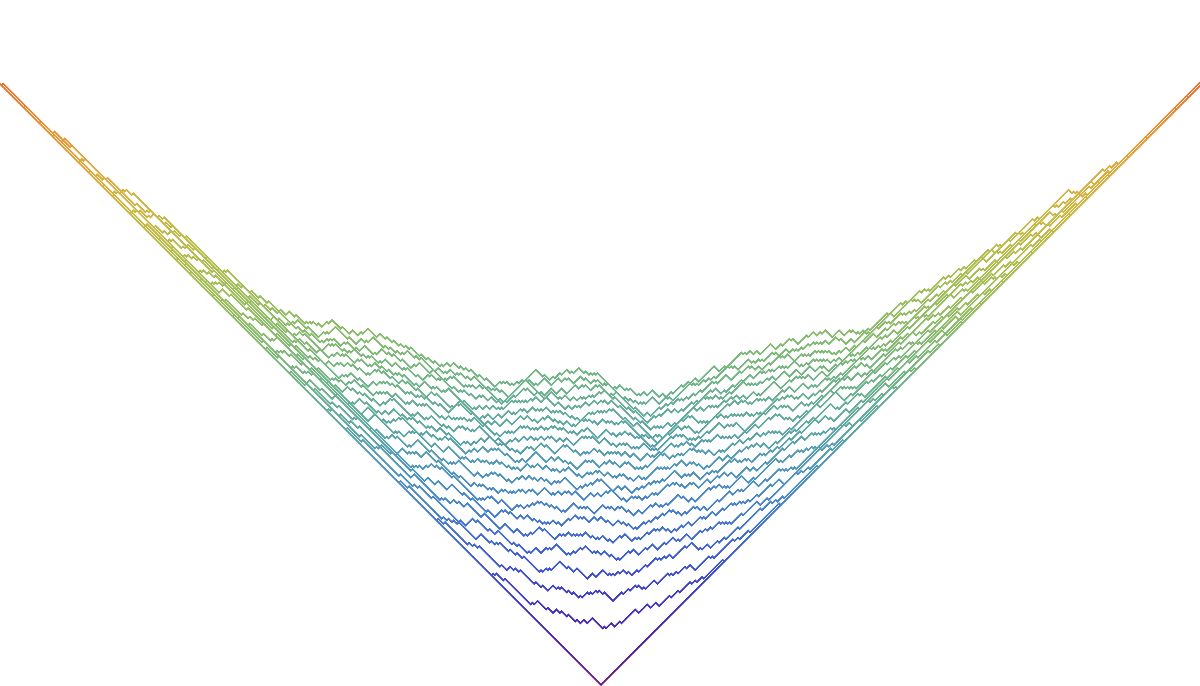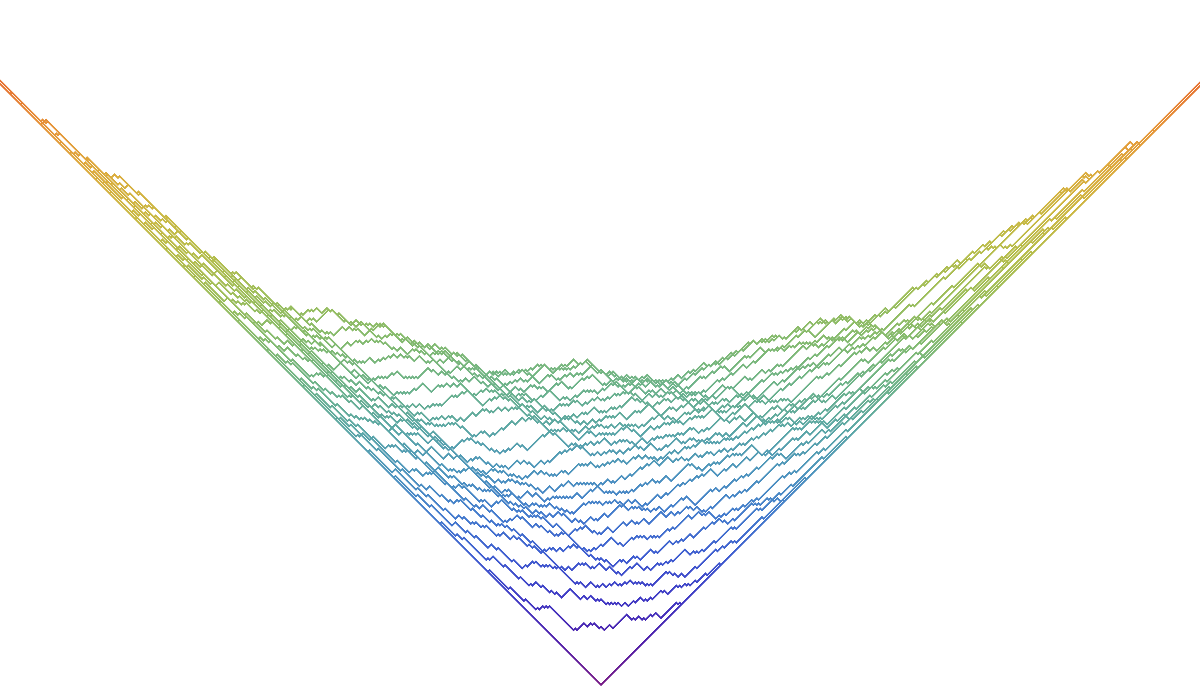Backwards evolution of TASEP

Hao Yu Li and Leonid Petrov

This is a simulation illustrating the main result from the paper in preparation .

Multitime distributions

In addition to the dynamical simulation (the movie below), we can generate joint pictures of the height function at different times. Here are the ones for TASEP:And for the backwards dynamics:Data file format for the movie

The file contains Mathematica-readable array of the form ${t_i,H(t_i)}$, where $t_i$ are the timestamps, and $H(t_i)$ is the array of the values of the TASEP height function.

code • (Main GitHub repo)

(note: parameters in the code might differ from the ones in simulation results below)
• (Code is available upon request)

simulation results

1. Movie • (data: 125 MB) • (graphics: 1.8 MB)
Movie. TASEP goes forward up to time $t=350$. Then the configuration evolves according to the backwards dynamics, all the way down to (almost) time zero. The timestamps are shown on top. At each time during the backwards dynamics, the distribution of the random height function coincides with the one of the TASEP at the specified time moment.references

1. L. Petrov, A. Saenz. In preparation (2019)